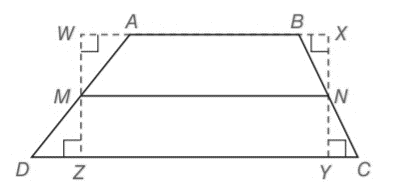Chapter 4.4, Problem 6EElementary Geometry For College St...

7th Edition
Alexander + 2 others
ISBN: 9781337614085

Solutions

Chapter
SectionElementary Geometry For College St...

7th Edition
Alexander + 2 others
ISBN: 9781337614085
Textbook Problem

In trapezoid ABCD, M N ¯ is the median. Without writing a formal proof explain why M N = 1 2 ( A B + D C ) .To determine

To explain:

Why MN=12(AB+DC).

Explanation

Consider the following trapezoid ABCD.

In a trapezoid ABCD, MN is the median.

We know that, the length of the median (m) of a trapezoid is the average of the lengths of the bases.

It means m=b

Still sussing out bartleby?

Check out a sample textbook solution.

See a sample solution

The Solution to Your Study Problems

Bartleby provides explanations to thousands of textbook problems written by our experts, many with advanced degrees!

Get Started

Let f(x) = {x21if2x1x3if1x2 Find (a) limx1 f(x) and limx1+ f(x). Is f continuous at x = 1? Explain.

Applied Calculus for the Managerial, Life, and Social Sciences: A Brief Approach

Use Exercise 52 to find x4exdx.

Single Variable Calculus: Early Transcendentals

01e2xdx= a) 2e2 1 b) `e2 1 c) 12e2 d) 12(e21)

Study Guide for Stewart's Single Variable Calculus: Early Transcendentals, 8th

Write as an iterated integral, where D is shown at the right.

Study Guide for Stewart's Multivariable Calculus, 8th